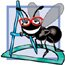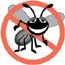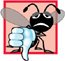# Function Definitions with Multiple Parameters

Chapters 35 presented classes containing simple functions that had at most one parameter. Functions often require more than one piece of information to perform their tasks. We now consider functions with multiple parameters.

(This item is displayed on pages 243 - 244 in the print version)

 ``` 1 // Fig. 6.3: GradeBook.h 2 // Definition of class GradeBook that finds the maximum of three grades. 3 // Member functions are defined in GradeBook.cpp 4 #include // program uses C++ standard string class 5 using std::string; 6 7 // GradeBook class definition 8 class GradeBook 9 { 10 public: 11 GradeBook( string ); // constructor initializes course name 12 void setCourseName( string ); // function to set the course name 13 string getCourseName(); // function to retrieve the course name 14 void displayMessage(); // display a welcome message 15 void inputGrades(); // input three grades from user 16 void displayGradeReport(); // display a report based on the grades 17 int maximum( int, int, int ); // determine max of 3 values 18 private: 19 string courseName; // course name for this GradeBook 20 int maximumGrade; // maximum of three grades 21 }; // end class GradeBook ```

Figure 6.4. GradeBook class defines function maximum.

(This item is displayed on pages 244 - 245 in the print version)

 ``` 1 // Fig. 6.4: GradeBook.cpp 2 // Member-function definitions for class GradeBook that 3 // determines the maximum of three grades. 4 #include 5 using std::cout; 6 using std::cin; 7 using std::endl; 8 9 #include "GradeBook.h" // include definition of class GradeBook 10 11 // constructor initializes courseName with string supplied as argument; 12 // initializes studentMaximum to 0 13 GradeBook::GradeBook( string name ) 14 { 15 setCourseName( name ); // validate and store courseName 16 maximumGrade = 0; // this value will be replaced by the maximum grade 17 } // end GradeBook constructor 18 19 // function to set the course name; limits name to 25 or fewer characters 20 void GradeBook::setCourseName( string name ) 21 { 22 if ( name.length() <= 25 ) // if name has 25 or fewer characters 23 courseName = name; // store the course name in the object 24 else // if name is longer than 25 characters 25 { // set courseName to first 25 characters of parameter name 26 courseName = name.substr( 0, 25 ); // select first 25 characters 27 cout << "Name "" << name << "" exceeds maximum length (25). " 28 << "Limiting courseName to first 25 characters. " << endl; 29 } // end if...else 30 } // end function setCourseName 31 32 // function to retrieve the course name 33 string GradeBook::getCourseName() 34 { 35 return courseName; 36 } // end function getCourseName 37 38 // display a welcome message to the GradeBook user 39 void GradeBook::displayMessage() 40 { 41 // this statement calls getCourseName to get the 42 // name of the course this GradeBook represents 43 cout << "Welcome to the grade book for " << getCourseName() << "! " 44 << endl; 45 } // end function displayMessage 46 47 // input three grades from user; determine maximum 48 void GradeBook::inputGrades() 49 { 50 int grade1; // first grade entered by user 51 int grade2; // second grade entered by user 52 int grade3; // third grade entered by user 53 54 cout << "Enter three integer grades: "; 55 cin >> grade1 >> grade2 >> grade3; 56 57 // store maximum in member studentMaximum 58 maximumGrade = maximum( grade1, grade2, grade3 ); 59 } // end function inputGrades 60 61 // returns the maximum of its three integer parameters 62 int GradeBook::maximum( int x, int y, int z ) 63 { 64 int maximumValue = x; // assume x is the largest to start 65 66 // determine whether y is greater than maximumValue 67 if ( y > maximumValue ) 68 maximumValue = y; // make y the new maximumValue 69 70 // determine whether z is greater than maximumValue 71 if ( z > maximumValue ) 72 maximumValue = z; // make z the new maximumValue 73 74 return maximumValue; 75 } // end function maximum 76 77 // display a report based on the grades entered by user 78 void GradeBook::displayGradeReport() 79 { 80 // output maximum of grades entered 81 cout << "Maximum of grades entered: " << maximumGrade << endl; 82 } // end function displayGradeReport ```

Figure 6.5. Demonstrating function maximum.

``` 1 // Fig. 6.5: fig06_05.cpp
4
5 int main()
6 {
9
10 myGradeBook.displayMessage(); // display welcome message
13 return 0; // indicate successful termination
14 } // end main
```

 ``` Welcome to the grade book for CS101 C++ Programming! Enter three integer grades: 86 67 75 Maximum of grades entered: 86 ```

 ``` Welcome to the grade book for CS101 C++ Programming! Enter three integer grades: 67 86 75 Maximum of grades entered: 86 ```

 ``` Welcome to the grade book for CS101 C++ Programming! Enter three integer grades: 67 75 86 Maximum of grades entered: 86 ```

Software Engineering Observation 6.4The commas used in line 58 of Fig. 6.4 to separate the arguments to function maximum are not comma operators as discussed in Section 5.3. The comma operator guarantees that its operands are evaluated left to right. The order of evaluation of a function's arguments, however, is not specified by the C++ standard. Thus, different compilers can evaluate function arguments in different orders. The C++ standard does guarantee that all arguments in a function call are evaluated before the called function executes.

Portability Tip 6.1Sometimes when a function's arguments are more involved expressions, such as those with calls to other functions, the order in which the compiler evaluates the arguments could affect the values of one or more of the arguments. If the evaluation order changes between compilers, the argument values passed to the function could vary, causing subtle logic errors.

Error-Prevention Tip 6.2If you have doubts about the order of evaluation of a function's arguments and whether the order would affect the values passed to the function, evaluate the arguments in separate assignment statements before the function call, assign the result of each expression to a local variable, then pass those variables as arguments to the function.

The prototype of member function maximum (Fig. 6.3, line 17) indicates that the function returns an integer value, that the function's name is maximum and that the function requires three integer parameters to accomplish its task. Function maximum's header (Fig. 6.4, line 62) matches the function prototype and indicates that the parameter names are x, y and z. When maximum is called (Fig. 6.4, line 58), the parameter x is initialized with the value of the argument grade1, the parameter y is initialized with the value of the argument grade2 and the parameter z is initialized with the value of the argument grade3. There must be one argument in the function call for each parameter (also called a formal parameter) in the function definition.

Notice that multiple parameters are specified in both the function prototype and the function header as a comma-separated list. The compiler refers to the function prototype to check that calls to maximum contain the correct number and types of arguments and that the types of the arguments are in the correct order. In addition, the compiler uses the prototype to ensure that the value returned by the function can be used correctly in the expression that called the function (e.g., a function call that returns void cannot be used as the right side of an assignment statement). Each argument must be consistent with the type of the corresponding parameter. For example, a parameter of type double can receive values like 7.35, 22 or 0.03456, but not a string like "hello". If the arguments passed to a function do not match the types specified in the function's prototype, the compiler attempts to convert the arguments to those types. Section 6.5 discusses this conversion.

Common Programming Error 6.1Declaring method parameters of the same type as double x, y instead of double x, double y is a syntax erroran explicit type is required for each parameter in the parameter list.

Common Programming Error 6.2Compilation errors occur if the function prototype, function header and function calls do not all agree in the number, type and order of arguments and parameters, and in the return type.

Software Engineering Observation 6.5A function that has many parameters may be performing too many tasks. Consider dividing the function into smaller functions that perform the separate tasks. Limit the function header to one line if possible.

To determine the maximum value (lines 6275 of Fig. 6.4), we begin with the assumption that parameter x contains the largest value, so line 64 of function maximum declares local variable maximumValue and initializes it with the value of parameter x. Of course, it is possible that parameter y or z contains the actual largest value, so we must compare each of these values with maximumValue. The if statement at lines 6768 determines whether y is greater than maximumValue and, if so, assigns y to maximumValue. The if statement at lines 7172 determines whether z is greater than maximumValue and, if so, assigns z to maximumValue. At this point the largest of the three values is in maximumValue, so line 74 returns that value to the call in line 58. When program control returns to the point in the program where maximum was called, maximum's parameters x, y and z are no longer accessible to the program. We'll see why in the next section.

There are three ways to return control to the point at which a function was invoked. If the function does not return a result (i.e., the function has a void return type), control returns when the program reaches the function-ending right brace, or by execution of the statement

```return;
```

If the function does return a result, the statement

```return expression;
```

evaluates expression and returns the value of expression to the caller.C++ How to Program (5th Edition)
ISBN: 0131857576
EAN: 2147483647
Year: 2004
Pages: 627